# Coloring random subgraphs

 Importance: Medium ✭✭
 Author(s): Bukh, Boris
 Subject: Graph Theory » Probabilistic Graph Theory
 Keywords: coloring random graph
 Posted by: mdevos on: June 18th, 2008

Ifis a graph and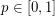, we letdenote a subgraph ofwhere each edge ofappears inwith independently with probability.

Problem   Does there exist a constantso that?

It is a classical result that the above problem has a positive answer whenis the complete graph. More generally, the lower boundis known.

It is easy to obtain the bound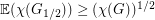, since we may imagine forming two random subgraphsofby putting each edge ofin eitherorindependently with probability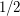. Then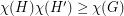and this gives the desired bound. A similar argument with three subgraphs shows that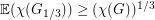, however these arguments all seem to require integer multiples, so the best known lower bound on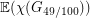of this form is.

## Bibliography

* indicates original appearance(s) of problem.

### No?

I haven't worked through the details yet, but what ifis the union ofand a sufficiently huge bipartite graph? Then, and by takinghuge enough, you can getas close to 2 as you like, forcingas small as you like.

EDIT: Whoo boy. Nevermind.